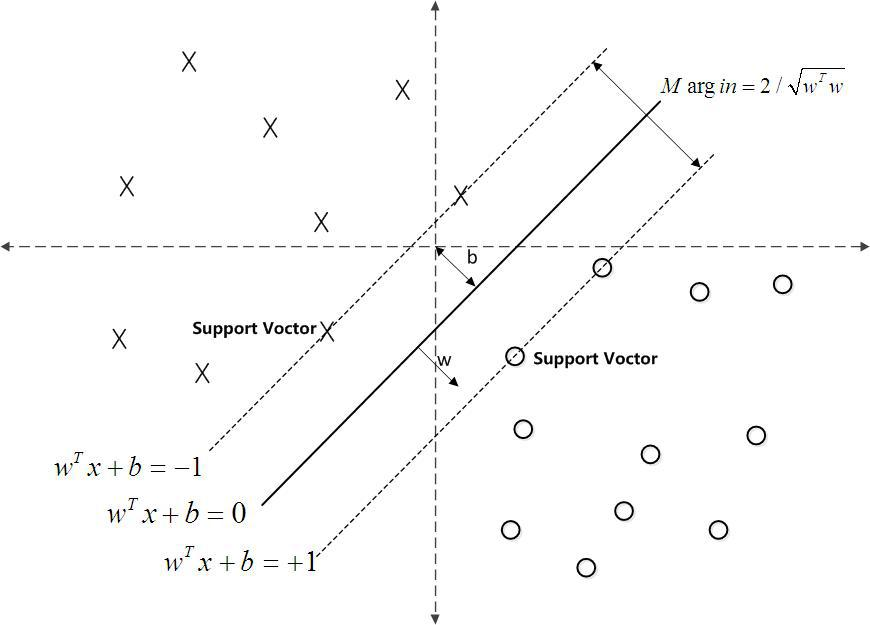# SVM

max

## 支持向量# 非线性情况

## 软间隔

$min\frac{1}{2}w^2+C\sum_{i=1}^{m}max(0, (1-y_i(w^Tx_i+b))$

$s.t. y_i(w^Tx_i+b)\ge1-\xi_i, i=1,2,…,m$

​ $\xi_i\ge0$

# 目标回归模型

SVR计算损失：允许存在偏差$\epsilon$, 仅当f(x)和y之间的差别大于偏差时才开始计算损失，即以f(x)为中心，构建了宽度为2ε的间隔带，当训练样本落入此间隔带，则认为预测正确。

alpha系数有多少个？